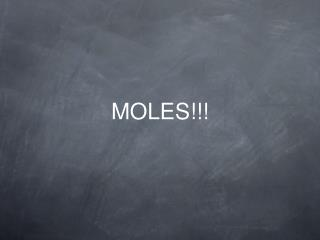DownloadDownload PresentationMOLES!!!

# MOLES!!!

Télécharger la présentation## MOLES!!!

- - - - - - - - - - - - - - - - - - - - - - - - - - - E N D - - - - - - - - - - - - - - - - - - - - - - - - - - -
##### Presentation Transcript

1. MOLES!!!

2. Atomic mass Review • the mass of an atom expressed in atomic mass units • is an average of the isotopes for that element • listed on the periodic table

3. What is a mole? • A mole is a counting number (like a dozen or a gross) • the number of atoms in exactly 12 g of carbon-12

4. Molar mass • the mass in grams of one mole of a substance (g/mol) • same number as the atomic mass but the units are different

5. Example • The average atomic mass of an Fe atom is 55.85 amu. • The molar mass of Fe is 55.85 g/mol

6. Conversions • To convert between moles and mass: • mass = # of moles x ___g/1 mol • use molar mass

7. Directions • Add the examples to your 3.4 notes • Copy the problems and solve the problems.

8. Example #1 • Find the mass of 3.000 moles of Fe.

9. Answer #1 3.000molFe x55.85g Fe = 167.6g Fe 1 mole Fe

10. Example #2 • Find the mass of 2.25 moles of Helium.

11. Answer #2 2.25 mol He x 4.00g He = 9.00 g He 1 mol He

12. Conversion #2 • Converting from mass to moles. Mass (g) x 1 mole = # moles molar mass (g)

13. Example #3 • How many moles of carbon in 49.5 g of carbon?

14. Answer #3 49.5 g C x 1 mol C = 4.12 mol C 12.01 g C

15. Avogadro’s number • the number of atoms/particles in one mole of a substance • = 6.022 x 1023 atoms/particles • 1 mole of anything is 6.022 x 1023 of that object

16. Conversion #3 • Determine the number of atoms from # moles # moles x 6.022 x 1023atoms = #atoms 1 mole

17. Example #4 • Determine the number of atoms in 4.00 moles of carbon. • The substance doesn’t matter if it is in moles.

18. Answer #4 • 2.41 x 1024 C atoms • 4.00 mole C x 6.022 x 1023 C atoms • 1 mole C

19. Conversion #4 • To convert number of atoms to moles • 9.05 x 1025 Fe atoms x 1 mol Fe • 6.022 x 1023 Fe atoms = 150. moles of Fe

20. Example #5 • Calculate the number of moles in 3.20 x 1023 atoms of iron. • The identity of the substances is not important when converting between moles & # of atoms.

21. Answer #5 • 0.531 moles of Fe • 3.20 x 1023Fe atoms x 1 mole Fe • 6.022 x 1023Fe atoms

22. Practice • Add to your notes: • Practice 1-4 p. 102 & practice 1-3 p. 103 • Copy problem; Show work; Include units in work & answer.

23. 0.850g H x 1 mol H = 0.842 molH • 1.01g H

24. Practice Problem Answers • P. 102: • 1. 238 g U 2. 1.2g U • 3. 0.842 mol H, 0.859g H • 4. 486.0 g Pb, • 0.01132 mol Pb

25. p. 103 • 1. 4.2 x 1023 atoms Fe • 2. 0.4796 mol Ag • 3. 0.58 mol Os

26. Homework • p. 104: 4,5,6-10,12,13